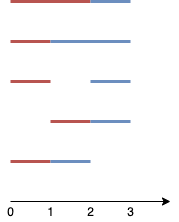# GeetCode Hub

Given `n` points on a 1-D plane, where the `ith` point (from `0` to `n-1`) is at `x = i`, find the number of ways we can draw exactly `k` non-overlapping line segments such that each segment covers two or more points. The endpoints of each segment must have integral coordinates. The `k` line segments do not have to cover all `n` points, and they are allowed to share endpoints.

Return the number of ways we can draw `k` non-overlapping line segments. Since this number can be huge, return it modulo `109 + 7`.

Example 1:```Input: n = 4, k = 2
Output: 5
Explanation:
The two line segments are shown in red and blue.
The image above shows the 5 different ways {(0,2),(2,3)}, {(0,1),(1,3)}, {(0,1),(2,3)}, {(1,2),(2,3)}, {(0,1),(1,2)}.```

Example 2:

```Input: n = 3, k = 1
Output: 3
Explanation: The 3 ways are {(0,1)}, {(0,2)}, {(1,2)}.
```

Example 3:

```Input: n = 30, k = 7
Output: 796297179
Explanation: The total number of possible ways to draw 7 line segments is 3796297200. Taking this number modulo 109 + 7 gives us 796297179.
```

Example 4:

```Input: n = 5, k = 3
Output: 7
```

Example 5:

```Input: n = 3, k = 2
Output: 1```

Constraints:

• `2 <= n <= 1000`
• `1 <= k <= n-1`

class Solution { public int numberOfSets(int n, int k) { } }